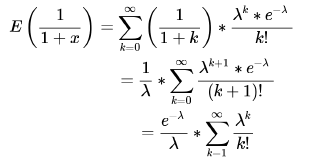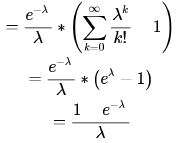Courses

# Probability MCQ - 1

## 20 Questions MCQ Test RRB JE for Computer Science Engineering | Probability MCQ - 1

Description
This mock test of Probability MCQ - 1 for Computer Science Engineering (CSE) helps you for every Computer Science Engineering (CSE) entrance exam. This contains 20 Multiple Choice Questions for Computer Science Engineering (CSE) Probability MCQ - 1 (mcq) to study with solutions a complete question bank. The solved questions answers in this Probability MCQ - 1 quiz give you a good mix of easy questions and tough questions. Computer Science Engineering (CSE) students definitely take this Probability MCQ - 1 exercise for a better result in the exam. You can find other Probability MCQ - 1 extra questions, long questions & short questions for Computer Science Engineering (CSE) on EduRev as well by searching above.
QUESTION: 1

### Suppose a fair six-sided die is rolled once. If the value on the die is 1, 2, or 3, the die is rolled a second time. What is the probability that the sum total of values that turn up is at least 6?

Solution:

Here our sample space consists of 3 + 3 * 6 = 21 events- (4), (5), (6), (1,1), (1,2) ... (3,6).
Favorable cases = (6), (1,5), (1,6), (2, 4), (2, 5), (2, 6), (3, 3), (3,4), (3,5), (3,6)
Required Probability = No. of favorable cases/Total cases = 10/21
But this is wrong way of doing. Because due to 2 tosses for some and 1 for some, individual probabilities are not the same. i.e., while (6) has 1/6 probability of occurrence, (1,5) has only 1/36 probability. So, our required probability
= 1/6 + (9 * 1/36) = 5/12

QUESTION: 2

### Box P has 2 red balls and 3 blue balls and box Q has 3 red balls and 1 blue ball. A ball is selected as follows: (i) select a box (ii) choose a ball from the selected box such that each ball in the box is equally likely to be chosen. The probabilities of selecting boxes P and Q are 1/3 and 2/3 respectively. Given that a ball selected in the above process is a red ball, the probability that it came from the box P is:

Solution:

The probability of selecting a red ball  = (1/3) * (2/5) + (2/3) * (3/4) = 2/15 + 1/2
= 19/30
Probability of selecting a red ball from box P = (1/3) * (2/5) = 2/15
Given that a ball selected in the above process is a red ball, the probability that it came from the box P is = (2/15) / (19/30) = 4/19

QUESTION: 3

### 10% of all email you receive is spam. Your spam filter is 90% reliable: that is, 90% of the mails it marks as spam are indeed spam and 90% of spam mails are correctly labelled as spam. If you see a mail marked spam by your filter, what is the probability that it really is spam?

Solution:

10% email are spam, i.e. 90% email are not spam 90% of mail marked as spam is spam, 10% mail marked as spam are not spam By Bayes theorem the probability that a mail marked spam is really a spam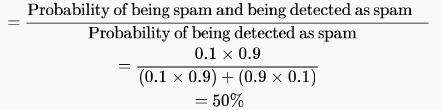QUESTION: 4

When a coin is tossed, the probability of getting a Head is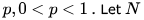. Let  be the random variable denoting the number of tosses till the first Head appears, including the toss where the Head appears. Assuming that successive tosses are independent, the expected value of N is

Solution: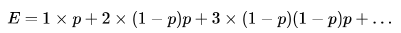multiply both side with  and subtract: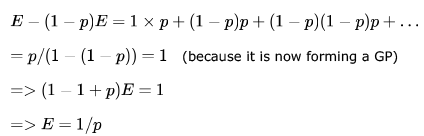QUESTION: 5

For each element in a set of size 2n, an unbiased coin is tossed. The 2n coin tosses are independent. An element is chosen if the corresponding coin toss was a head. The probability that exactly n elements are chosen is

Solution:

ways of getting n heads out of 2n tries = 2nCn
probability of getting exactly n heads and n tails = (1/2n)(1/2n) number of ways = 2nCn/4n

QUESTION: 6

Suppose three coins are lying on a table, two of them with heads facing up and one with tails facing up. One coin is chosen at random and flipped. What is the probability that after the flip the majority of the coins(i.e., at least two of them) will have heads facing up?

Solution:

table has 3 coins with H,H,T facing up.
now, probability of chosing any coin is 1/3 , as we can chose any of three coins.
Case A: 1st coin :  either H or T can come.
so, HHT THT possible.only HHT is favourable.
which gives (1/3)*(1/2)= 1/6
Case B: 2nd coin :  either H or T can come.
so, HHT HTT possible.only HHT is favourable.
which gives (1/3)*(1/2)= 1/6
case C: 3rd coin : Table already contains two H's so, whatever comes is favourable.
which gives (1/3)*1 = 1/3
summing up total gives 1/6 + 1/6 + 1/3 = 2/3

QUESTION: 7

A random bit string of length n is constructed by tossing a fair coin n times and setting a bit to 0 or 1 depending on outcomes head and tail, respectively. The probability that two such randomly generated strings are not identical is:

Solution:

suppose there are k places within n bit string where mismatch has occoured probability of this occouring is nCk(prob. of mismatch)k(prob. of match)(n - k) = nCk(1/2)k(1/2)(n-k) = nCk(1/2)n
k can range from 1 to n hence required probability sum(nCk(1/2)n) where k ranges from 1 to n
hence (1/2n)(2n - 1)

Alternatively Probability of matching at given place 1/2
there are n places hence probability of matching 1/(2n)
hence probability of mismatch 1 - 1/(2n)

QUESTION: 8

Karan tells truth with probability 1/3 and lies with probability 2/3. Independently, Arjun tells truth with probability 3/4 and lies with probability 1/4. Both watch a cricket match. Arjun tells you that India won, Karan tells you that India lost. What probability will you assign to India's win?

Solution:

Probability of India win =1/2
Probability of India lost=1/2
If really India wins, then Karan lies i.e.=2/3 and Arjun tells truth=3/4
Now prob. of Karan lies  and Arjun tells truth=2/3 * 3/4=1/2
Now prob. of Arjun lies  and Karan tells truth=1/4 * 1/3=1/12
so, by Bayes theorem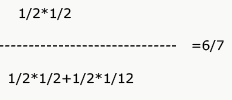*Answer can only contain numeric values
QUESTION: 9

Suppose that a shop has an equal number of LED bulbs of two different types. The probability of an LED bulb lasting more than  hours given that it is of Type 0.7 is , and given that it is of Type 2 is 0.4. The probability that an LED bulb chosen uniformly at random lasts more than 100 hours is _________.

Solution:

Suppose that a shop has an equal number of LED bulbs of two different types.  ==> Therefore
Probability of Taking Type 1 Bulb => 0.5
Probability of Taking Type 2 Bulb => 0.5
The probability of an LED bulb lasting more than 100 hours given that it is of Type 1 is 0.7, and given that it is of Type 2 is 0.4.
Prob(100+ | Type1) => 0.7
Prob(100+| Type2) => 0.4
Prob(100+) => Prob(100+ | Type1) * Prob(Type1) + Prob(100+ | Type2) * Prob(Type2)
= 0.7 * .5 + .4 * .5
= 0.55

SIMPLY-
TYPE-1 bulbs ===>let total =10 then 7 are going for 100 years
TYPE-2 bulbs====>let total=10 then 4 are going for 100 years
TOTAL==>20 out of which 11 are going for 100 years ..THEREFORE probability=11/20=.55

QUESTION: 10

P and Q are considering to apply for a job. The probability that P applies for the job is 1/4, the probability that P applies for the job given that Q applies for the job is 1/2., and the probability that Q applies for the job given that P applies for the job is 1/3. Then the probability that P does not apply for the job given that Q does not apply for this job is

Solution:

Let,
P = P applies for the job
Q = Q applies for the job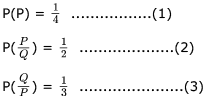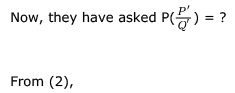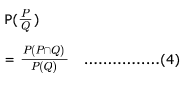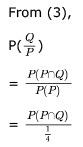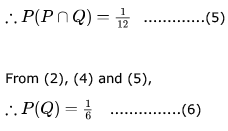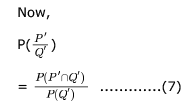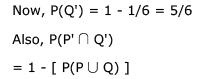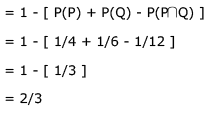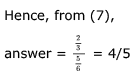QUESTION: 11

Let A and B be any two arbitrary events, then, which one of the following is true?

Solution:

(a) is true only if events are independent.
(b) is true only if events are mutually exclusive i.e.
(c) is false everywhere
(d) is always true as  P(A U B) = P(A) + P(B) — P(A ∩ B)*Answer can only contain numeric values
QUESTION: 12

Each of the nine words in the sentence  " The quick brown fox jumps over the lazy dog"  is written on a separate piece of paper. These nine pieces of paper are kept in a box. One of the pieces is drawn at random from the box. The length of the word drawn is _____________. (The answer should be rounded to one decimal place.)

Solution:

Each of the nine words have equal probability. So, expected length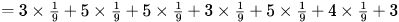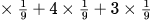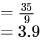QUESTION: 13

An examination paper has 150 multiple choice questions of one mark each, with each question having four choices. Each incorrect answer fetches -0.25 marks. Suppose 1000 students choose all their answers randomly with uniform probability.The sum total of the expected marks obtained by all these students is

Solution:

Probability of choosing the correct option = 1/4
Probability of choosing a wrong option = 1/3
So, expected mark for a question for a student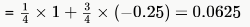Expected mark for a student for 150 questions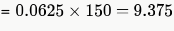So, sum total of the expected marks obtained by all 1000 students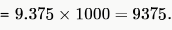QUESTION: 14

Assume that you are flipping a fair coin, i.e. probability of heads or tails is equal. Then the expected number of coin flips required to obtain two consecutive heads for the first time is.

Solution:

Let the expected number of coin flips be . The case analysis goes as follows:
a. If the first flip is a tails, then we have wasted one flip. The probability of this event is 1/2 and the total number of flips required is X + 1.

b. If the first flip is a heads and second flip is a tails, then we have wasted two flips. The probability of this event is 1/4 and the total number of flips required is X + 2 as the same scenario as beginning is there even after 2 tosses.
c. If the first flip is a heads and second flip is also heads, then we are done. The probability of this event is 1/4 and the total number of flips required is 2.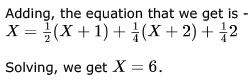Thus, the expected number of coin flips for getting two consecutive heads is 6.

QUESTION: 15

A bag contains  balls of the following colors: 8 red, 4 blue, 2 green, 1 black, and 1 white. Anisha picks a ball randomly from the bag, and messages Babu its color using a string of zeros and ones. She replaces the ball in the bag, and repeats this experiment, many times. What is the minimum expected length of the message she has to convey to Babu per experiment?

Solution:

using static huffman compression you can encode the more common colours in fewer bits than the rare colours, that being the case on can expect that common colours will usually be chosen.
eg:
red    1
blue   01
green  001
white  0001
black  0000

on average from 16 draws there will be

8 reds   = 8 bits
4 blues  = 8 bits
2 greens = 6 bits
1 white  = 4 bits
1 black  = 4 bits

for a total of 30/16 = 15/8 bits on average

*Answer can only contain numeric values
QUESTION: 16

A fair die is tossed two times. the probability that 2nd toss results in value greater than first toss is ?

Solution:

total outcomes = 36
expected outcomes={(1,2),(1,3),(1,4),(1,5),(1,6),(2,3),(2,4),(2,5),(2,6),(3,4),(3,5),(3,6),(4,5),(4,6),(5,6)}=15
hence Probability=15/36=5/12

= 0.41666

*Answer can only contain numeric values
QUESTION: 17

If a random variable X has a Poisson distribution with mean 5, then the expectation E[(X+2)2] equals ___.

Solution:

In Poisson distribution :
Mean  =  Variance  as  n is large and p is small
And we know :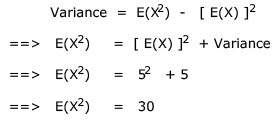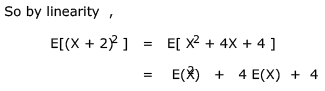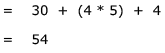Hence 54 should be the right answer..

QUESTION: 18

In a multi-user operating system on an average, 20 requests are made to use a particular resource per hour. The arrival of requests follows a Poisson distribution. The probability that either one, three or five requests are made in 45 minutes is given by :

Solution:

20 request in 1 hour.. so we can expect 15 request in 45 minutes...
So, lemda = 15.. (expected value)
poission distribution formula: f(x, lemda) = p(X = x) = (lemda ^ x * e ^ - lemda)  / x!
Therefore p(one request) + p(3 request) + p(5 request)
= p(1; 15) + p(3; 15) + p(5; 15)
= 6.9 * 10^3 * e ^ -15..
= 6.9*103*e-15 = 6.9*103*e5*e-20 = 1.02*106*e-20..  Ans is (B)

QUESTION: 19

Suppose p is the number of cars per minute passing through a certain road junction between 5 PM and 6 PM, and p has a Poisson distribution with mean 3. What is the probability of observing fewer than 3 cars during any given minute in this interval?

Solution:

Poisson Probability Density Function (with mean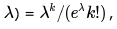We have to sum the probability density function for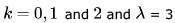(thus finding the cumulative mass function)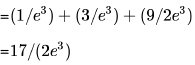QUESTION: 20

Suppose X is distributed as Poisson with mean λ. Then E(1/(X + 1)) is

Solution: